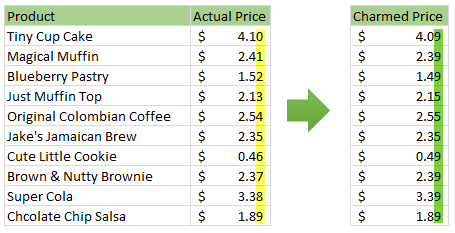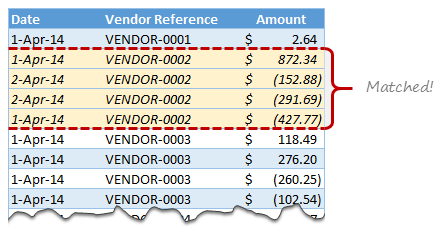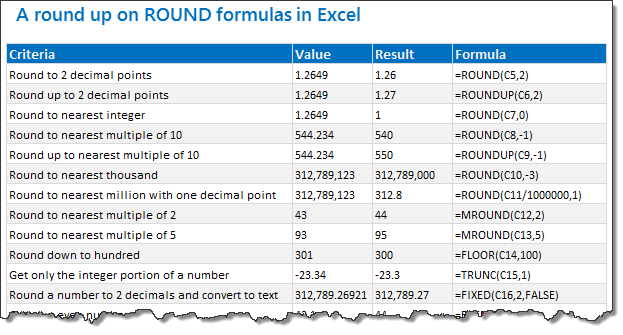# All articles with 'round' Tag

## Rounding time to nearest minute or quarter hour etc. [formulas]

Published on Jun 26, 2017 in Excel HowtosThe other day, I was building a spreadsheet to calculate FTE (full time equivalent) for staff based on hours worked on various days in a fortnight. While building the spreadsheet, I came across an interesting problem. Rounding Time to nearest minute.  We can’t use ROUND() or MROUND() to round time as these formulas aren’t designed to work with time values. Although time values are technically decimal, rounding time to nearest minute (or quarter hour etc.) can be tricky when usual round formulas. Let me share a few formulas to round time to nearest point.

Let’s say you have a time value (either user input or calculated) in cell A1.

Use below formulas to round time in A1.

Continue »

## Charmed Price Problem

Published on Oct 6, 2014 in Excel HowtosHere is a charming little problem to kick start your day.

Lets say you run a cute little bakery around the corner. Since you want your prices to look charming, you have a policy to round them down or up based on below rule.

If the price ends with 0, 1 or 2 cents, round it down to 9 cents.

If the price ends with 3, 4 or 5 cents, round it up to 5 cents.

If the price ends with 6, 7, 8 or 9 cents, round it up to 9 cents.

For example,

So how do you round to nearest charmed price? You could do it manually. But you would rather bake a few more of those Tiny Cup Cakes than waste time rounding the prices. So you want an automatic way to round prices. This is where Excel helps.

Continue »

## Matching transactions using formulas [Accounting]

Published on Jun 6, 2014 in Excel Howtos, Learn ExcelImagine you are the head of Accounts Receivable department at a large company. Drab, I know, But humor me and imagine.

Now, every month you get a transaction report.

And you want to know which numbers are matching up.

i.e, if your company gave Vendor-0002 \$872.34 on 1st of April, 2014 and your received below payments from them subsequently,

• \$427.77 on 1st April
• \$152.88 on 2nd April
• \$291.69 on 2nd April

Then you consider the account matched since the total received is same as total payable.(427.77 + 152.88 + 291.69 = 872.34).

Continue »

## 18.2 Tips on Rounding numbers using Excel Formulas

Published on Sep 28, 2012 in Excel Howtos, Learn ExcelLets talk round numbers today.I have 18.2 tips for you on round numbers.

We can use a variety of formulas to round numbers in Excel depending on the situation. We have ROUND, ROUNDUP, ROUNDDOWN, MROUND, INT, TRUNC, CEILING, FLOOR, FIXED, EVEN, ODD and few more. To know how to use all these formulas and how to round numbers based on any criteria, just read on.

Continue »

## How to Round and Sort Data using Excel Formulas?

Published on Jul 6, 2009 in Excel Howtos, Learn ExcelCheryl asks via e-mail, “I was wondering if you could help me figure out how to combine the round formula with the rank formula? I need to first round all the numbers and then rank them.”

Of course we can solve this by simply using array formulas. Curious? Find out more by reading the rest of this post.

Continue »

### Get FREE Excel & Power-BI Newsletter

One email per week with Excel and Power BI goodness. Join 100,000+ others and get it free.